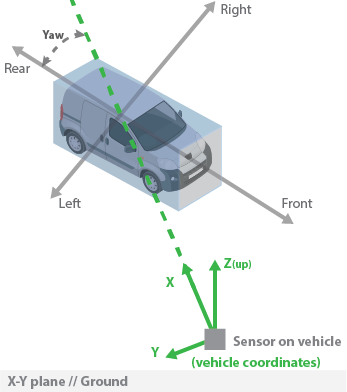# projectedCuboidTo3D

Compute 3-D cuboid from 2-D projected cuboid

Since R2022b

## Syntax

``cuboid = projectedCuboidTo3D(projectedCuboid,worldHeight,monoCam)``
``cuboid = projectedCuboidTo3D(___,FrontFace=face)``

## Description

example

````cuboid = projectedCuboidTo3D(projectedCuboid,worldHeight,monoCam)` computes the 3-D cuboid from the 2-D projected cuboid `projectedCuboid`, the height data of the objects contained in the projected cuboids `worldHeight`, and the camera parameters for the sensor that collected the images `monoCam`.```
````cuboid = projectedCuboidTo3D(___,FrontFace=face)` specifies which side of each 2-D projected cuboid to align with the front of the object, in addition to the input arguments from the previous syntax.```

## Examples

collapse all

Specify the intrinsic parameter for a forward-facing, monocular camera sensor mounted on an ego vehicle. First, specify the focal length, the principal point of the image plane, and the output image size, in pixels.

```focalLength = [309.4362 344.2161]; principalPoint = [318.9034 257.5352]; imageSize = [480 640];```

Then specify the position of the camera. Position the camera 1.5 meters above the ground.

`height = 1.5;`

Save the intrinsic parameters as a `cameraIntrinsics` object. Define a monocular camera sensor using the intrinsic camera parameters and the position of the camera.

```camIntrinsics = cameraIntrinsics(focalLength,principalPoint,imageSize); sensor = monoCamera(camIntrinsics,height);```

Define a pair of 2-D projected cuboids, specified as a 2-by-8 matrix.

```projectedCuboidLabel = [302 573 255 202 376 533 255 202; 192 542 86 77 247 530 86 77]; ```

Define the height of the objects contained within them in world units (m).

`objectHeight = 1.5; `

Estimate 3-D cuboids from the 2-D projected cuboids using the heights of the objects contained within them and the previously defined parameters of the monocular camera sensor.

`cuboid = projectedCuboidTo3D(projectedCuboidLabel,objectHeight,sensor)`
```cuboid = 2×9 2.3209 -1.1070 0.7500 0.5550 1.9126 1.5000 0 0 -90.0000 2.3209 0.4231 0.7500 0.4125 0.6450 1.5000 0 0 -90.0000 ```

## Input Arguments

collapse all

2-D projected cuboids, specified as an M-by-8 matrix. M is the number of cuboids. Each row of the matrix defines the front and rear rectangular faces of a 2-D projected cuboid in the form [x1 y1 w1 h1 x2 y2,w2 h2], where [x1 y1] and [x2 y2] specify the upper-left coordinates of the front and rear rectangular faces, and [w1 h1] and [w2 h2] specify the width and height of the corresponding faces respectively.

Heights of objects in meters, specified as an M-by-1 vector or a real scalar. M is the number of objects.

Camera parameters, specified as a `monoCamera` object. The object contains the intrinsic and extrinsic parameters of the camera used to capture the images.

Front face of the projected cuboids, specified as `"rear"`, `"front"`, `"left"`, `"right"`, or an M-by-1 string array in which each element is `"rear"`, `"front"`, `"left"`, or `"right"`. M is the number of cuboids. This argument specifies which side of each projected 2-D cuboid is aligned with the front of the object contained within it.

• `"rear"` — Face of the 2-D projected cuboid furthest from the observer.

• `"front"` — Face of the 2-D projected cuboid closest to the observer.

• `"left"` — Face of the 2-D projected cuboid to the left from the perspective of the observer.

• `"right"` — Face of the 2-D projected cuboid to the right from the perspective of the observer.

If you specify this argument as a scalar value, the function applies that value to all input projected cuboids. If you specify this argument as an M-by-1 string array, the function applies the value of each element to the corresponding cuboid in `projectedCuboid`. Use this argument if the front of one or more detected objects is aligned with the left, right, or front of the cuboid that contains it. For example, if a detected vehicle is facing the sensor, specify the face value for that cuboid as `"front"`.

Data Types: `char` | `string`

## Output Arguments

collapse all

3-D cuboids, returned as an M-by-9 matrix. M is the number of 3-D cuboids. Each row of the matrix defines the position, size, and orientation of a 3-D cuboid in the form [xctr yctr zctr xlen ylen zlen xrot yrot zrot]. The function assumes `0` degrees of roll and pitch, and only returns the yaw angle.

• Position — The center of the cuboid, in the vehicle coordinate system, is represented by xctr, yctr, zctr

• Size — The length of the cuboid in the x-, y- and z- directions is represented by xlen, ylen, and zlen, respectively.

• Orientation — The pitch, roll, and yaw angles, in degrees, are represented by xrot, yrot, zrot, respectively.

This diagram shows how the function determines the yaw angle (zrot) based on the angle between the face of the sensor that detected the cuboid and the selected front face of the cuboid. In this case, the value of `face` is "front".## Version History

Introduced in R2022b